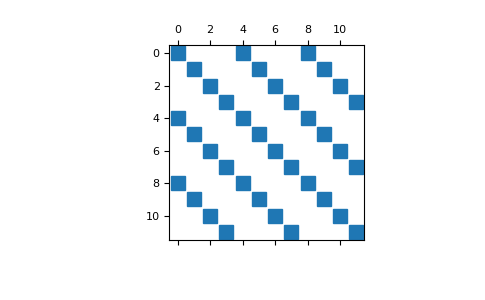# discretize.utils.inverse_3x3_block_diagonal¶

discretize.utils.inverse_3x3_block_diagonal(a11, a12, a13, a21, a22, a23, a31, a32, a33, return_matrix=True, **kwargs)[source]

Invert a set of 3x3 matricies from vectors containing their elements.

Parameters
a11, a12, …, a33(n_blocks) numpy.ndarray

Vectors which contain the corresponding element for all 3x3 matricies

return_matrixbool, optional
• True: Returns the sparse block 3x3 matrix M (default).

• False: Returns the vectors containing the elements of each matrix’ inverse.

Returns
(3 * n_blocks, 3 * n_blocks) scipy.sparse.coo_matrix or list of (n_blocks)

numpy.ndarray. If return_matrix = False, the function will return vectors b11, b12, b13, b21, b22, b23, b31, b32, b33. If return_matrix = True, the function will return the block matrix M.

Notes

The elements of a 3x3 matrix A are given by:

\begin{align}\begin{aligned}A = \begin{bmatrix} a_{11} & a_{12} & a_{13} \\a_{21} & a_{22} & a_{23} \\a_{31} & a_{32} & a_{33} \end{bmatrix}\end{aligned}\end{align}

For a set of 3x3 matricies, the elements may be stored in a set of 9 distinct vectors $$\mathbf{a_{11}}$$, $$\mathbf{a_{12}}$$, …, $$\mathbf{a_{33}}$$. For each matrix, inverse_3x3_block_diagonal ouputs the vectors containing the elements of each matrix’ inverse; i.e. $$\mathbf{b_{11}}$$, $$\mathbf{b_{12}}$$, …, $$\mathbf{b_{33}}$$ where:

\begin{align}\begin{aligned}A^{-1} = B = \begin{bmatrix} b_{11} & b_{12} & b_{13} \\b_{21} & b_{22} & b_{23} \\b_{31} & b_{32} & b_{33} \end{bmatrix}\end{aligned}\end{align}

For special applications, we may want to output the elements of the inverses of the matricies as a 3x3 block matrix of the form:

\begin{align}\begin{aligned}M = \begin{bmatrix} D_{11} & D_{12} & D_{13} \\D_{21} & D_{22} & D_{23} \\D_{31} & D_{32} & D_{33} \end{bmatrix}\end{aligned}\end{align}

where $$D_{ij}$$ are diagonal matrices whose non-zero elements are defined by vector $$\mathbf{b_{ij}}$$. Where n is the number of matricies, the block matrix is sparse with dimensions (3n, 3n).

Examples

Here, we define four 3x3 matricies and reorganize their elements into 9 vectors a11, a12, …, a33. We then examine the outputs of the function inverse_3x3_block_diagonal when the argument return_matrix is set to both True and False.

>>> from discretize.utils import inverse_3x3_block_diagonal
>>> import numpy as np
>>> import scipy as sp
>>> import matplotlib.pyplot as plt


Define four 3x3 matricies, and organize their elements into nine vectors

>>> A1 = np.random.uniform(1, 10, (3, 3))
>>> A2 = np.random.uniform(1, 10, (3, 3))
>>> A3 = np.random.uniform(1, 10, (3, 3))
>>> A4 = np.random.uniform(1, 10, (3, 3))
>>> [[a11, a12, a13], [a21, a22, a23], [a31, a32, a33]] = np.stack(
...     [A1, A2, A3, A4], axis=-1
... )


Return the elements of their inverse and validate

>>> b11, b12, b13, b21, b22, b23, b31, b32, b33 = inverse_3x3_block_diagonal(
...     a11, a12, a13, a21, a22, a23, a31, a32, a33, return_matrix=False
... )
>>> Bs = np.stack([[b11, b12, b13],[b21, b22, b23],[b31, b32, b33]])
>>> B1, B2, B3, B4 = Bs.transpose((2, 0, 1))

>>> np.linalg.inv(A1)
array([[ 0.20941584,  0.18477151, -0.22637147],
[-0.06420656, -0.34949639,  0.29216461],
[-0.14226339,  0.11160555,  0.0907583 ]])
>>> B1
array([[ 0.20941584,  0.18477151, -0.22637147],
[-0.06420656, -0.34949639,  0.29216461],
[-0.14226339,  0.11160555,  0.0907583 ]])


We can also return this as a sparse matrix with block diagonal inverse

>>> M = inverse_3x3_block_diagonal(
...     a11, a12, a13, a21, a22, a23, a31, a32, a33
... )
>>> plt.spy(M)
>>> plt.show()